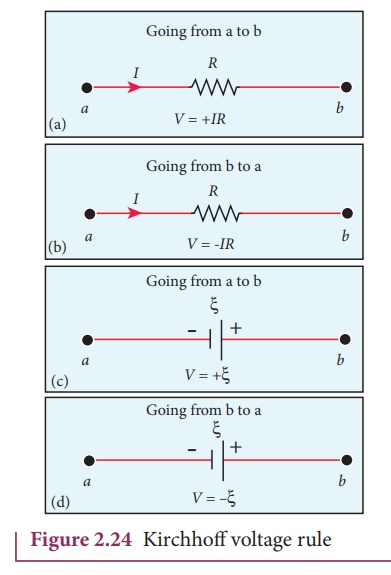Home | | Physics 12th Std | KirchhoffŌĆÖs Second rule (Voltage rule or Loop rule)

# KirchhoffŌĆÖs Second rule (Voltage rule or Loop rule)

It states that in a closed circuit the algebraic sum of the products of the current and resistance of each part of the circuit is equal to the total emf included in the circuit.

KirchhoffŌĆÖs Second rule (Voltage rule or Loop rule)

It states that in a closed circuit the algebraic sum of the products of the current and resistance of each part of the circuit is equal to the total emf included in the circuit. This rule follows from the law of conservation of energy for an isolated system (The energy supplied by the emf sources is equal to the sum of the energy delivered to all resistors).The product of current and resistance is taken as positive when the direction of the current is followed. Suppose if the direction of current is opposite to the direction of the loop, then product of current and voltage across the resistor is negative. It is shown in Figure 2.24 (a) and (b). The emf is considered positive when proceeding from the negative to the positive terminal of the cell. It is shown in Figure 2.24 (c) and (d).Kirchhoff voltage rule has to be applied only when all currents in the circuit reach a steady state condition (the current in various branches are constant).

EXAMPLE 2.21

The following figure shows a complex network of conductors which can be divided into two closed loops like ACE and ABC. Apply KirchoffŌĆÖs voltage rule.Solution

Thus applying KirchoffŌĆÖs second law to the closed loop EACE

I1R1 + I2R2 + I3R3 = ╬Š

and for the closed loop ABCA

I4R4 + I5R5-I2R2= 0

EXAMPLE 2.22

Calculate the current that flows in the 1ŌĆå╬® resistor in the following circuit.SolutionWe can denote the current that flows from 9V battery as I1 and it splits into I2 and I1  ŌĆō I2 in the junction according KirchoffŌĆÖs current rule (KCR). It is shown below.

Now consider the loop EFCBE and apply KVR, we get

1I2 + 3I1 + 2I1 = 9

5I1 + I2 = 9 (1)

Applying KVR to the loop EADFE, we get

3 (I1 ŌĆō I2 ) ŌĆō 1I2 = 6

3I1 ŌĆō 4I2 = 6 (2)

Solving equation (1) and (2), we get

I1 = 1.83 A and I2 = -0.13 A

It implies that the current in the 1 ohm resistor flows from F to E.

Tags : Explanation, Formulas, Solved Example Problems , 12th Physics : Current Electricity
Study Material, Lecturing Notes, Assignment, Reference, Wiki description explanation, brief detail
12th Physics : Current Electricity : KirchhoffŌĆÖs Second rule (Voltage rule or Loop rule) | Explanation, Formulas, Solved Example Problems